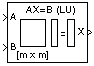Documentation

# LU Solver

Solve AX=B for X when A is square matrix

## Library

Math Functions / Matrices and Linear Algebra / Linear System Solvers

`dspsolvers`## Description

The LU Solver block solves the linear system AX=B by applying LU factorization to the M-by-M matrix at the A port. The input to the B port is the right side M-by-N matrix, B. The M-by-N matrix output X is the unique solution of the equations.

The block treats length-M unoriented vector input to the input port B as an M-by-1 matrix.

## Algorithm

The LU algorithm factors a row-permuted variant (Ap) of the square input matrix A as

`${A}_{p}=LU$`

where L is a lower triangular square matrix with unity diagonal elements, and U is an upper triangular square matrix.

The matrix factors are substituted for Ap in

`${A}_{p}X={B}_{p}$`

where Bp is the row-permuted variant of B, and the resulting equation

`$LUX={B}_{p}$`

is solved for X by making the substitution Y = UX, and solving two triangular systems.

`$\begin{array}{l}LY={B}_{p}\hfill \\ UX=Y\hfill \end{array}$`

## Examples

See Linear System Solvers for an example that uses the LU Solver block.

## Supported Data Types

• Double-precision floating point

• Single-precision floating point

 Autocorrelation LPC DSP System Toolbox Cholesky Solver DSP System Toolbox LDL Solver DSP System Toolbox Levinson-Durbin DSP System Toolbox LU Factorization DSP System Toolbox LU Inverse DSP System Toolbox QR Solver DSP System Toolbox

See Linear System Solvers for related information.

Watch now